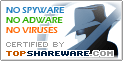# Equation Grapher

SNAPSHOT

Equation grapher is an easy-to-use software for 2D function graphing.

Windows AllPlatform :
\$0Price :
1.23 MBFile Size :
ScreenshotScreenshot :Popularity :Equation grapher is an easy-to-use software for 2D function graphing. 2D function graphs can be plotted in Cartesian and polar coordinate systems.Equation grapher is an easy-to-use software for 2D function graphing. 2D function graphs can be plotted in Cartesian and polar coordinate systems.Equation grapher is an easy-to-use software for 2D function graphing. 2D function graphs can be plotted in Cartesian and polar coordinate systems

• Visual Matrix
Matrix calculator for matrix operation and matrix decomposition.
• Complex Grapher
Complex Grapher is a graphing calculator to create a graph of complex function.
• Visual Data
Scientific surface data and volume data visualization software.
• Visual Optim
Visual Optim is a math program for linear and nonlinear programming.
• PR Submitter
Submit your press releases to more than 210 sites.
• Calc 3D
calculator for vectors, matrices, complex numbers and quaternions
• ProKalc
Scientific/Financial/Geometry Calculator
• Guzinta Math
Free Windows and Macintosh desktop lesson apps.
• CalculationLaboratory
Mathematical program
• CurvFit2
A nonlinear curve fitting program: Lorentzian, Sine, Power, Exponential series
• Matrix Calculator
Perform matrix algebra calculations.
• Robot4
Robotic Arm Movement program; finds angles ... came from NASA.
• 9CalFree
Calculator software with fancy interface
• Equation Wizard
Automatically solves algebraic equations of any order written in any form
• Science Helper For Ms Word
It's a software for making scientific graphs.